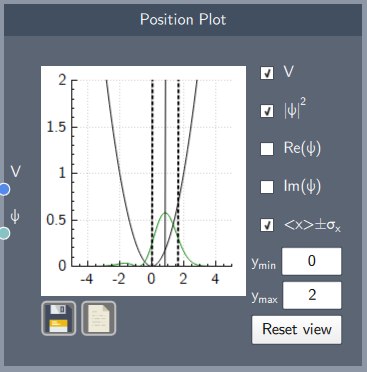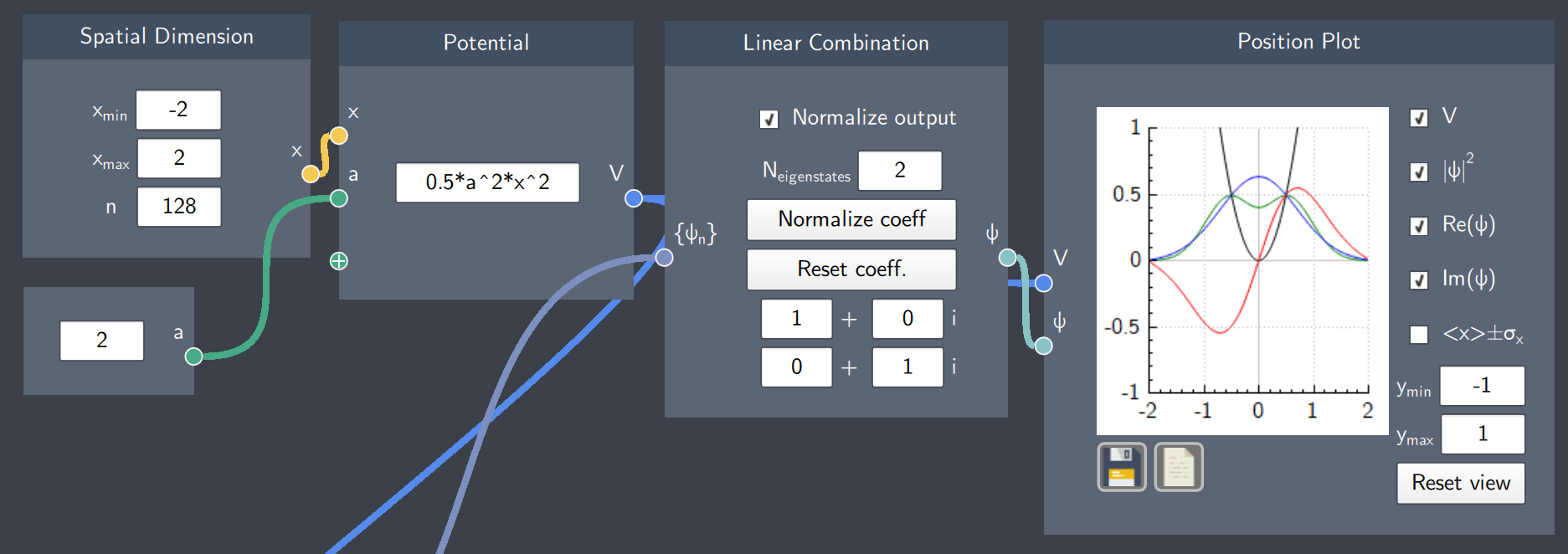# Position Plot

## Description

The Position Plot node plots the potential function, probability density, the real and imaginary parts of a wave function, and the expectation value of position (\langle x \rangle) and its standard deviation.## Input

The node has the following inputs:

• Potential ($V$): The Potential node where the potential function is defined
• Wave Function ($\psi$): Any analytically defined or linearly-combined wave function

## Content

This plot consists of check-boxes corresponding to different functions, which can be selected to visualize them.

The different check-boxes/functions from top to bottom (with required inputs in parenthesis) are:

• Show/Hide the potential (V)
• Show/Hide the probability density of the state ($\psi$)
• Show/Hide the real part of the state ($\psi$)
• Show/Hide the imaginary part of the state ($\psi$)
• Show/Hide the expectation value of the position and its standard deviation

Additionally, the $y$-axis limits can be set to any limits and reset to the original scale.  The plot can be saved and the data can be exported as a $.csv$ file.

## Output

The output of the node is the visualization in the plot based on the inputs and selection of the check-boxes.

## Example

In the example below, the harmonic oscillator potential has been plotted along with a superposition state (linear combination of the first two eigenstates of the harmonic oscillator) as selected in the check-boxes. The probability density, real part and imaginary part of the state are denoted by the blue, green and red curves respectively.